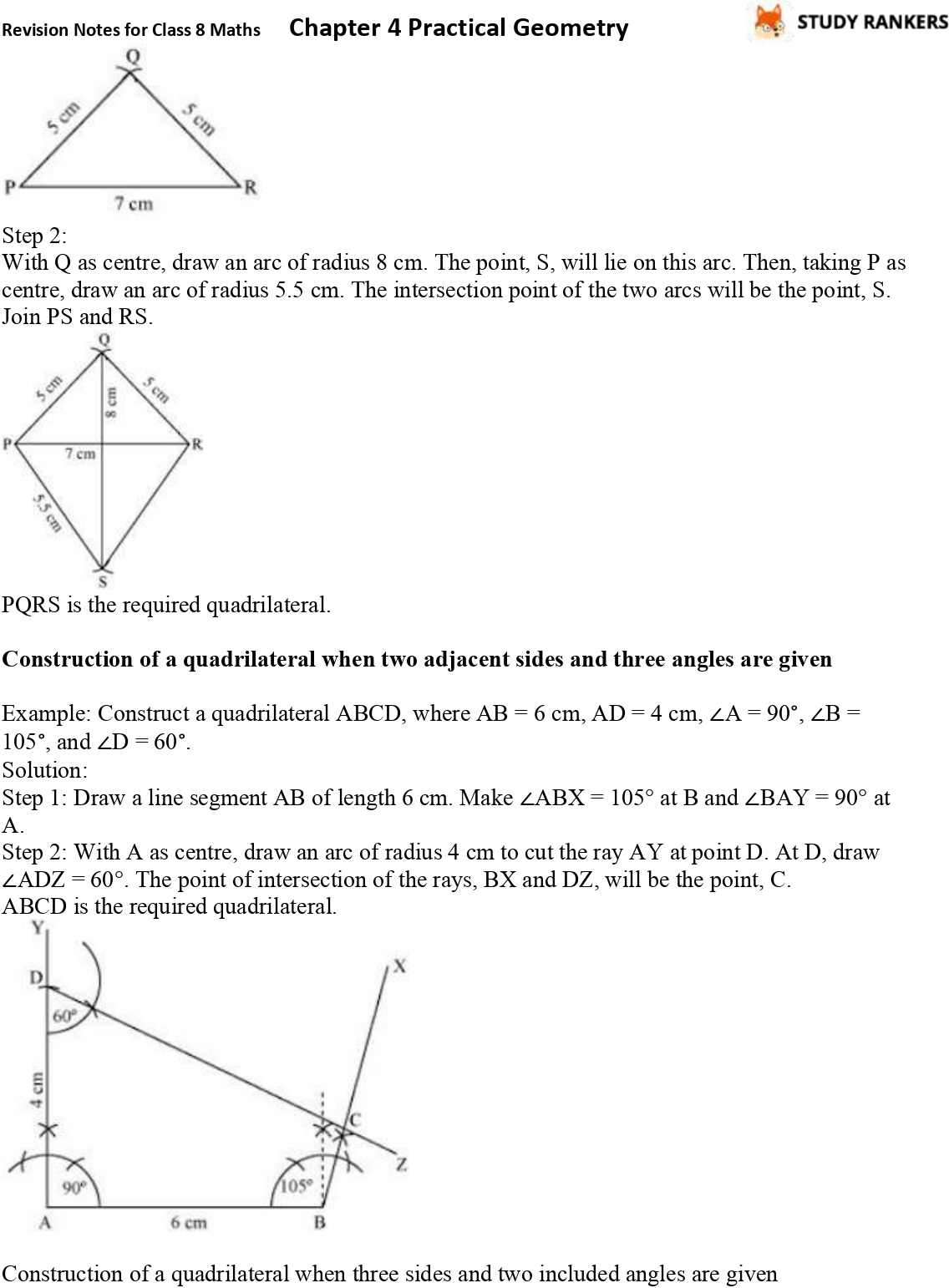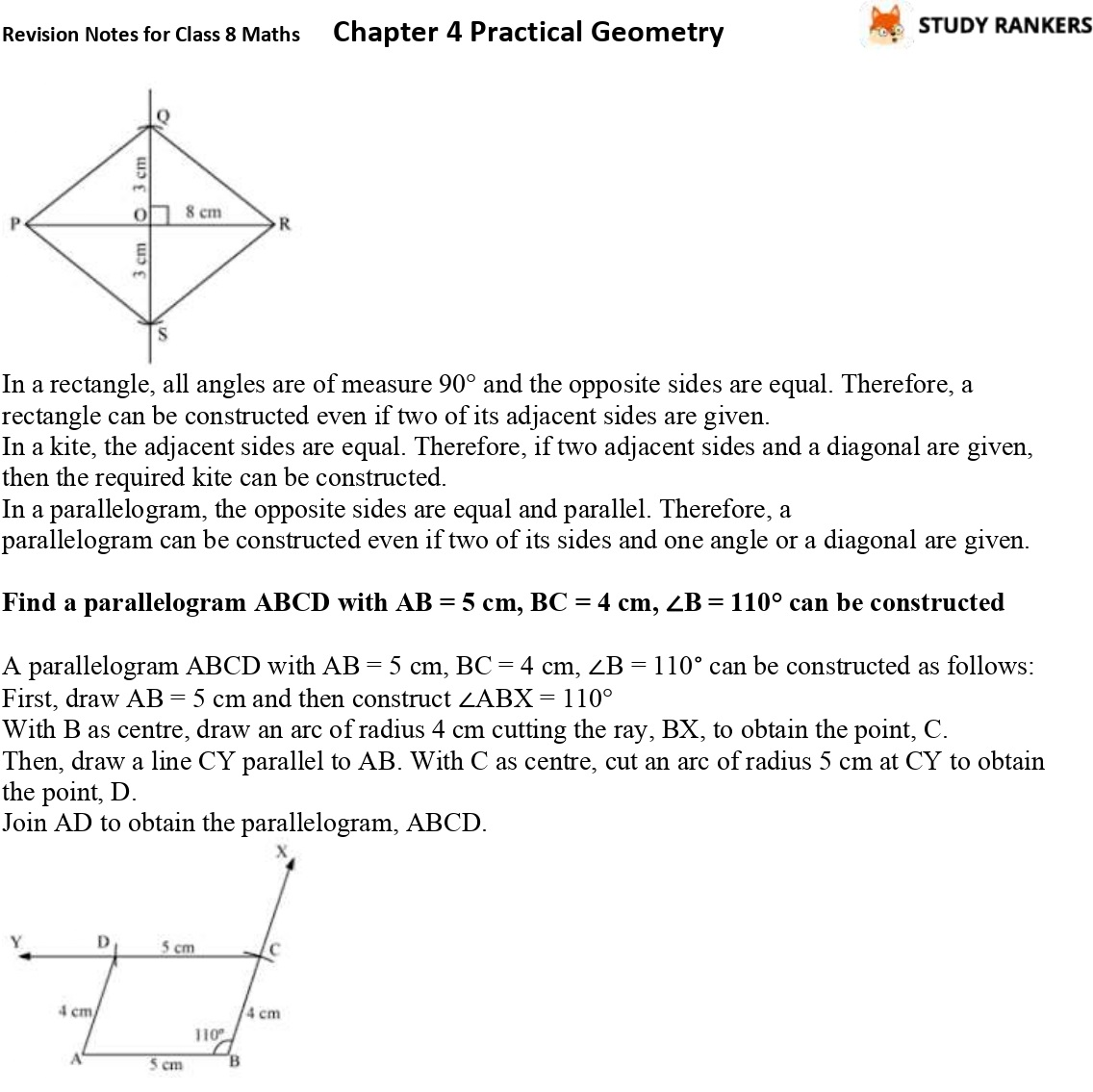## CBSE Revision Notes for Class 8 Chapter 4 Practical Geometry

Revision Notes for Class 8 Maths Chapter 4 is provided here which will be helpful in understanding what given in the chapter. Through the help of Chapter 4 Practical Geometry Revision Notes, you can prepare for examinations well and also enhance your knowledge. These Class 8 Revision Notes are prepared by Studyrankers expert faculty which will explain every important detail of the chapter so you can cover whole syllabus.
Topics in the chapter:
• Construction of a quadrilateral when four sides and a diagonal are given
• Construction of a quadrilateral when two diagonals and three sides are given
• Construction of a quadrilateral when two adjacent sides and three angles are given
• Construction of a quadrilateral when three sides and two included angles are given
• Construction of special quadrilaterals such as square, rhombus, rectangle, kite, and parallelogram can be done by using their properties.
• Find a parallelogram ABCD with AB = 5 cm, BC = 4 cm, ∠B = 110° can be constructed..